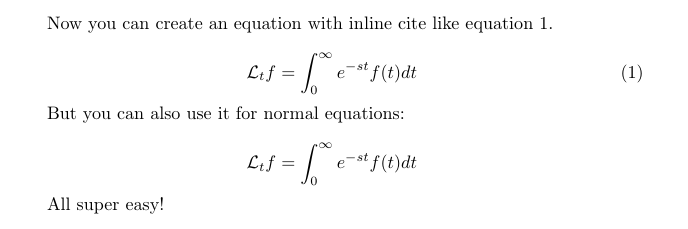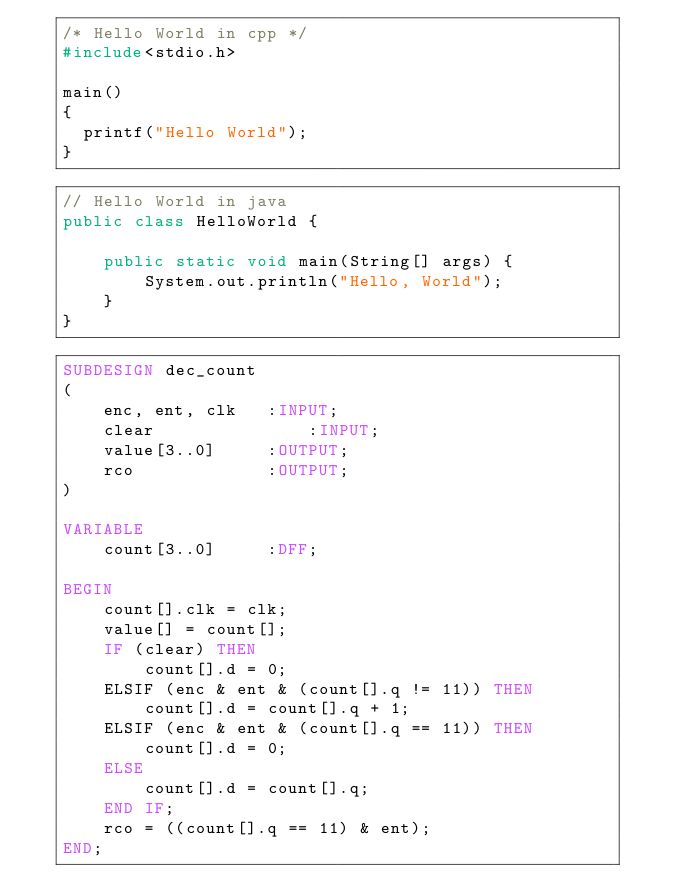# 智障的 LaTeX 入门教程（四）

Posted by Towdium on September 2, 2017

## 值与命令

#### 调用格式

1
\afunction[optional]{compulsory1}{compulsory2}


1
\afunction{compulsory1}{compulsory2}


#### 值定义

1
2
3
4
5
6
\documentclass{article}
\newcommand{\name}{Towdium the Stupid}

\begin{document}
This guy call himself \name .
\end{document}


1
This guy call himself Towdium the Stupid.


#### 命令定义

1
2
3
4
\begin{equation}
\mathcal{L}_t{f}=\int^\infty _0 e^{-st}f(t)dt
\label{eq}
\end{equation}


1
$$\mathcal{L}_t{f}=\int^\infty _0 e^{-st}f(t)dt$$


1
2
3
4
5
6
7
8
9
10
11
12
13
14
15
16
17
18
19
20
21
22
\documentclass{article}
\usepackage{ifthen, amsmath}

\newcommand{\eq}[]{
\ifthenelse{\equal{#1}{}}{ %
\begin{equation*} #2 \end{equation*} %
}{ %
\begin{equation} #2 \label{#1} \end{equation} %
}
}

\begin{document}
Now you can create an equation with inline cite like equation \ref{eq}.

\eq[eq]{\mathcal{L}_t{f}=\int^\infty _0 e^{-st}f(t)dt}

But you can also use it for normal equations:

\eq{\mathcal{L}_t{f}=\int^\infty _0 e^{-st}f(t)dt}

All super easy!
\end{document}1
2
3
4
if #1 == '':
\begin{equation*} #2 \end{equation*}
else:
\begin{equation} #2 \label{#1} \end{equation}


## 文件导入

1
2
3
4
5
6
7
8
9
10
11
12
\documentclass{article}
\usepackage{ifthen, amsmath, filecontents}

\newcommand{\name}{Towdium the Stupid}
\end{filecontents}

\begin{document}
This guy call himself \name .
\end{document}


## 带你折腾 listings

1
2
3
4
5
6
7
8
9
10
11
12
13
14
15
16
17
18
19
20
21
22
23
24
25
26
27
28
29
30
31
32
33
34
35
36
37
38
39
40
41
42
43
44
45
46
47
48
49
50
51
52
53
54
55
56
57
58
59
60
61
62
63
64
65
66
67
68
69
70
71
72
73
74
75
76
77
78
79
80
81
82
83
84
85
86
87
88
89
90
91
92
93
94
95
96
97
98
99
100
101
102
103
104
105
106
107
108
109
110
111
112
113
114
115
116
\documentclass{article}
\usepackage{color, listings}

\definecolor{mygray}{rgb}{0.5,0.5,0.4}
\definecolor{mygreen}{rgb}{0,0.7,0.5}
\definecolor{myorange}{rgb}{1.0,0.4,0}
\definecolor{mylilas}{rgb}{0.8,0.3,1.0}

\lstdefinestyle{mybase} {
breaklines=true,
showstringspaces=false,
basicstyle=\small\tt,
frame=single,
xleftmargin=20pt,
xrightmargin=20pt,
numbersep=9pt,
numberstyle={\color{black} \footnotesize \sf},
stringstyle=\color{myorange},
keywordstyle=\color{mygreen},
}

\lstdefinestyle{mycpp} {
style=mybase,
language=C++
}

\lstdefinestyle{myjava} {
style=mybase,
language=java
}

\lstdefinestyle{myahdl} {
style=mybase,
language = ahdl,
keywordstyle=\color{mylilas},
}

\lstdefinelanguage{ahdl}{
morekeywords={
AND, ASSERT, BEGIN, BIDIR, BiTS, BURIED,
CASE, CLIQUE, CONNECTED_PINS, CONSTANT,
DEFAULTS, DEFINE, DESIGN, DEVICE, DIV,
ELSE, ELSEIF, END, FOR, FUNCTION,
GENERATE, GND, HELP_ID,
IF, INCLUDE, INPUT, IS, LOG2,
MACHINE, MOD, NAND, NODE, NOR, NOT,
OF, OPTIONS, OR, OTHERS, OUTPUT,
PARAMETERS, REPORT, RETURNS,
SEGMENTS, SEVERITY, STATES, SUBDESIGN,
TABLE, THEN, TITLE, TO, TRI_STATE_NODE,
VARIABLE, VCC, WHEN, WITH, XNOR, XOR,
EXP, FLOOR, GLOBAL, JEFFE, JKFF,
LATCH, LCELL, MCELL, MEMORY, OPNDRN,
SOFT, SRFFE, SRFF, TFFE, TFF, TRI,
WIRE, X
},
sensitive=false,
morecomment=[l]{--},
morecomment=[s]{\%}{\%},
tabsize = 4
}

\begin{document}

\begin{lstlisting}[style = mycpp]
/* Hello World in cpp */
#include<stdio.h>

main()
{
printf("Hello World");
}
\end{lstlisting}

\begin{lstlisting}[style = myjava]
// Hello World in java
public class HelloWorld {

public static void main(String[] args) {
System.out.println("Hello, World");
}
}
\end{lstlisting}

\begin{lstlisting}[style = myahdl]
SUBDESIGN dec_count
(
enc, ent, clk	:INPUT;
clear				:INPUT;
value[3..0]		:OUTPUT;
rco				:OUTPUT;
)

VARIABLE
count[3..0]		:DFF;

BEGIN
count[].clk = clk;
value[] = count[];
IF (clear) THEN
count[].d = 0;
ELSIF (enc & ent & (count[].q != 11)) THEN
count[].d = count[].q + 1;
ELSIF (enc & ent & (count[].q == 11)) THEN
count[].d = 0;
ELSE
count[].d = count[].q;
END IF;
rco = ((count[].q == 11) & ent);
END;
\end{lstlisting}

\end{document}## 结

Fun with LaTeX!